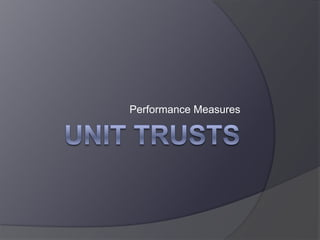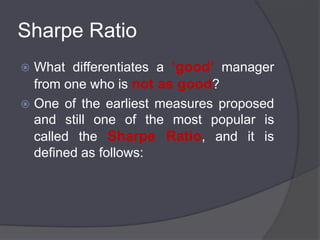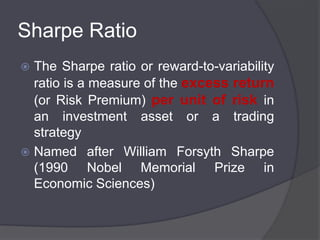Successfully reported this slideshow.

# Unit Trusts Mesurements(Aangepas)×

1 of 22
1 of 22

# Unit Trusts Mesurements(Aangepas)

## More Related Content

### Unit Trusts Mesurements(Aangepas)

1. 1. Unit Trusts<br />Performance Measures<br />
2. 2. Sharpe Ratio<br />What differentiates a ‘good’ manager from one who is not as good? <br />One of the earliest measures proposed and still one of the most popular is called the Sharpe Ratio, and it is defined as follows:<br />
3. 3. Sharpe Ratio<br />The Sharpe ratio or reward-to-variability ratio is a measure of the excess return (or Risk Premium) per unit of risk in an investment asset or a trading strategy <br />Named after William Forsyth Sharpe (1990 Nobel Memorial Prize in Economic Sciences)<br />
4. 4. Sharpe Ratio<br />Sharpe Ratio = (Rp – rf ) / σ p<br />where:<br />Rp= the return of the portfolio,<br />rf = the risk-free rate, usually chosen as a 3 month BA rate<br />σ p = the volatility of the portfolio , usually calculated as the standard deviation of returns.<br />Risk<br />Premuim<br />
5. 5. Sharpe Ratio<br />The basic idea is that an investment with a higher Sharpe ratio is a better investment. A higher ratio indicates more return per unit of risk assumed by the manager<br />
6. 6. Sortino Ratio<br />The Sortino ratio is closely related to the Sharpe ratio. It compares the return of a portfolio with a chosen Minimum Acceptable Return (which could be the risk free rate but need not be), and divides it by the downside semi-standard deviation, which measures only the volatility of returns below the MAR:<br />
7. 7. Sortino Ratio<br />Downside deviation<br />Similar to the loss standard deviation except the downside deviation considers only returns that fall below a defined Minimum Acceptable Return (MAR) rather then the arithmetic mean<br />
8. 8. Sortino Ratio<br />Sortinoratio = Rp – MAR / σDDMAR<br />Rp = the return of the portfolio<br />MAR = Minimum Acceptable Return<br />σDD= Downside deviation of return.<br />
9. 9. Sortino Ratio<br />Where the Sharpe Ratio measures return per unit of total risk the Sortino Ratio measures the return per measure of downside risk. <br />Thus what is the chance a manager’s portfolio will go below the MAR which is usually the risk free rate of return.<br />
10. 10. Information Ratio<br />IR attempts to measure not just the excess return to a benchmark, but also how consistent that performance is. <br />Is a manager beating the benchmark by a little every period, or a lot in a few particular periods? <br />Most investors would prefer the former, and the IR measures this degree of consistency. <br />
11. 11. Information Ratio<br />To define the IR we first have to define a related concept called the tracking error. <br />The tracking error is defined as the volatility, or standard deviation, of excess return. The excess return is calculated as the unit trust’s return minus the benchmark return <br />The IR is then defined as the excess return divided by the tracking error.<br />
12. 12. Information Ratio<br />Information Ratio = (Rp-Ri) / σ p-i<br />σ p-i =Tracking error <br />Rp = Unit Trust Return<br />Ri = Benchmark return<br />
13. 13. Example<br />The following information is given for 2 Unit trusts A and B<br />The benchmark for these unit trusts is the JSE ALSI<br />The AVERAGE risk free rate rf = 5%.<br />The Minimum Acceptable Return (MAR) = 5% = rf<br />
14. 14. Example<br />
15. 15. Example<br />Sharpe ratio (5 Year)<br />Sharpe Ratio A = (1.69 – 5) / 12.74<br />=-0.26<br />Sharpe Ratio B = (6.02-5) / 7.75<br /> = 0.13<br />
16. 16. Example<br />According to the Sharpe Ratio Unit Trust B delivers better return per unit of risk than Unit Trust A.<br />
17. 17. Example<br />= A minus Average risk free rate<br />= Excess return if negative otherwise 0<br />
18. 18. Example<br />SortinoRatio (5 Year)<br />SortinoRatio A = (1.69-5) / 9.78<br /> = -0.34<br />SortinoRatio B = (6.02-5) / 5.6 <br /> = 0.18<br />
19. 19. Example<br />According to the Sortino Ratio Unit Trust A has a better chance of going below the MAR which in this case is the Average Risk Free Rate<br />
20. 20. Example<br />= A minus ALSI<br />
21. 21. InformatonRatio (5 Year)<br />Information Ratio A = (-2.14) / 6.98<br /> = -0.31<br />Information Ratio B = 0.52 / 2.62<br /> = 0.2<br />
22. 22. Example<br />According to the Information Ratio Unit Trust B performs better than the benchmark (JSE ALSI) on a more consistent basis than Unit Trust A.<br />